# RSOZ

Given and Stell (Refs 1 and 2) provided exact Ornstein-Zernike relations for two-phase random media based on the original work of Madden and Glandt (Refs 3 and 4). For a two-species system, for the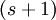$(s+1)$ replicated system one has (see Eq.s 2.7 --2.11 Ref. 2):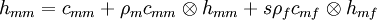$h_{mm} = c_{mm} + \rho_m c_{mm} \otimes h_{mm} + s\rho_f c_{mf} \otimes h_{mf}$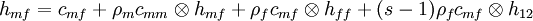$h_{mf} = c_{mf} + \rho_m c_{mm} \otimes h_{mf} + \rho_f c_{mf} \otimes h_{ff} + (s-1) \rho_f c_{mf} \otimes h_{12}$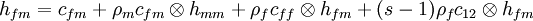$h_{fm} = c_{fm} + \rho_m c_{fm} \otimes h_{mm} + \rho_f c_{ff} \otimes h_{fm} + (s-1) \rho_f c_{12} \otimes h_{fm}$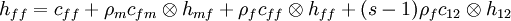$h_{ff} = c_{ff} + \rho_m c_{fm} \otimes h_{mf} + \rho_f c_{ff} \otimes h_{ff} + (s-1) \rho_f c_{12} \otimes h_{12}$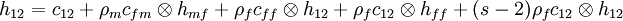$h_{12} = c_{12} + \rho_m c_{fm} \otimes h_{mf} + \rho_f c_{ff} \otimes h_{12} + \rho_f c_{12} \otimes h_{ff} + (s-2) \rho_f c_{12} \otimes h_{12}$

In the limit of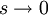$s \rightarrow 0$ these equations from the replica Ornstein-Zernike (ROZ)equations (see Eq.s 2.12 --2.16 Ref. 2):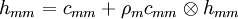$h_{mm} = c_{mm} + \rho_m c_{mm} \otimes h_{mm}$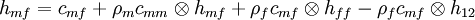$h_{mf} = c_{mf} + \rho_m c_{mm} \otimes h_{mf} + \rho_f c_{mf} \otimes h_{ff} - \rho_f c_{mf} \otimes h_{12}$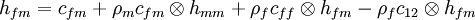$h_{fm} = c_{fm} + \rho_m c_{fm} \otimes h_{mm} + \rho_f c_{ff} \otimes h_{fm} - \rho_f c_{12} \otimes h_{fm}$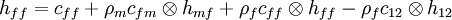$h_{ff} = c_{ff} + \rho_m c_{fm} \otimes h_{mf} + \rho_f c_{ff} \otimes h_{ff} - \rho_f c_{12} \otimes h_{12}$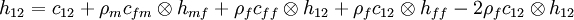$h_{12} = c_{12} + \rho_m c_{fm} \otimes h_{mf} + \rho_f c_{ff} \otimes h_{12} + \rho_f c_{12} \otimes h_{ff} -2 \rho_f c_{12} \otimes h_{12}$

When written in the percolation terminology' where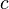$c$ terms connected and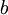$b$ blocking are adapted from the language of percolation theory.$h_{mm} = c_{mm} + \rho_m c_{mm} \otimes h_{mm}$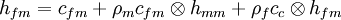$h_{fm} = c_{fm} + \rho_m c_{fm} \otimes h_{mm} + \rho_f c_c \otimes h_{fm}$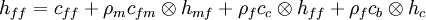$h_{ff} = c_{ff} + \rho_m c_{fm} \otimes h_{mf} + \rho_f c_c \otimes h_{ff} + \rho_f c_b \otimes h_c$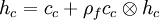$h_c = c_c + \rho_f c_c \otimes h_c$

where the direct correlation function is split into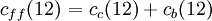$\left.c_{ff}(12)\right. = c_c (12) + c_b (12)$

and the total correlation function is also split into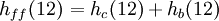$\left.h_{ff}(12)\right.= h_c (12) + h_b(12)$

where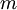$m$ denotes the matrix and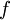$f$ denotes the fluid. The blocking function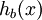$h_b(x)$ accounts for correlations between a pair of fluid particles blocked" or separated from each other by matrix particles. IMPORTANT NOTE: Unlike an equilibrium mixture, there is only one convolution integral for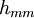$h_{mm}$ because the structure of the medium is unaffected by the presence of fluid particles.

• Note: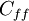$C_{ff}$ (Madden and Glandt)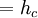$=h_c$ (Given and Stell)
• Note: fluid:$f$ (Madden and Glandt), 1' (Given and Stell)
• Note: matrix:$m$ (Madden and Glandt), `0' (Given and Stell)

At very low matrix porosities, i.e. very high densities of matrix particles, the volume accessible to fluid particles is divided into small cavities, each totally surrounded by a matrix. In this limit, the function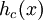$h_c (x)$ describes correlations between fluid particles in the same cavity and the function$h_b(x)$ describes correlations between particles in different cavities.

## Polydisperse systems

For a polydisperse fluid, composed of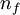$n_f$ components, in a polydisperse matrix, composed of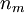$n_m$ components, written in matrix form in Fourier space (see Eq. 18 of Ref. 5):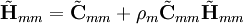$\tilde{\mathbf H}_{mm} = \tilde{\mathbf C}_{mm} + \rho_m \tilde{\mathbf C}_{mm} \tilde{\mathbf H}_{mm}$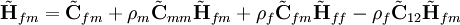$\tilde{\mathbf H}_{fm} = \tilde{\mathbf C}_{fm} + \rho_m \tilde{\mathbf C}_{mm} \tilde{\mathbf H}_{fm} + \rho_f \tilde{\mathbf C}_{fm} \tilde{\mathbf H}_{ff} - \rho_f \tilde{\mathbf C}_{12} \tilde{\mathbf H}_{fm}$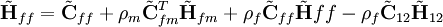$\tilde{\mathbf H}_{ff} = \tilde{\mathbf C}_{ff} + \rho_m \tilde{\mathbf C}_{fm}^T \tilde{\mathbf H}_{fm} + \rho_f \tilde{\mathbf C}_{ff} \tilde{\mathbf H}{ff} - \rho_f \tilde{\mathbf C}_{12} \tilde{\mathbf H}_{12}$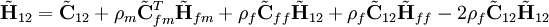$\tilde{\mathbf H}_{12} = \tilde{\mathbf C}_{12} + \rho_m \tilde{\mathbf C}_{fm}^T \tilde{\mathbf H}_{fm} + \rho_f \tilde{\mathbf C}_{ff} \tilde{\mathbf H}_{12} + \rho_f \tilde{\mathbf C}_{12} \tilde{\mathbf H}_{ff} -2 \rho_f \tilde{\mathbf C}_{12} \tilde{\mathbf H}_{12}$

Note: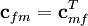${\mathbf c}_{fm} = {\mathbf c}_{mf}^T$ and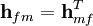${\mathbf h}_{fm} = {\mathbf h}_{mf}^T$.